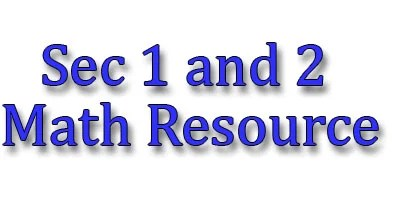## Algebra

Introduction to Algebra – Interactive Lesson –   https://deck.toys/a/7y7goMFbE

Algebraic Manipulation – Interactive Lesson – https://deck.toys/a/mkI6qK9-N

Algebra – Simultaneous Equation  (Intermediate) Online Quiz

https://www.transum.org/software/SW/Starter_of_the_day/Students/Simultaneous_Equations.asp?Level=3

https://www.transum.org/software/SW/Starter_of_the_day/Students/Simultaneous_Equations.asp?Level=6

Algebra – Simultaneous Equation  (Word Problems) Online Quiz – https://www.transum.org/software/SW/Starter_of_the_day/Students/Simultaneous_Equations.asp?Level=5

## Numbers

Real Numbers – Interactive Lesson – https://deck.toys/a/7kHw1ztyV

## Trigonometry

Pythagorus Theorem – Interactive Lesson – https://deck.toys/a/7koVV1r-N